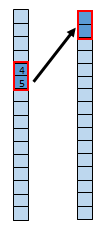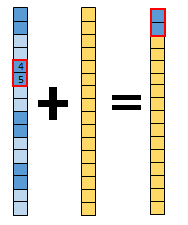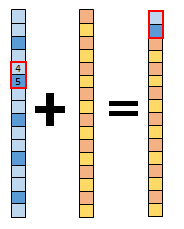# Transpose Bits function

## Overview

The Transpose Bit function unpacks the bits of the input pixel and maps them to specified bit locations in the output pixel. The purpose of this function is to manipulate multiple bit sequences from an input, such as the Landsat 8 quality band products.

## Notes

### Bit Pattern

The bit pattern dictates which bits within the quality band will be rearranged. Landsat 8 has a quality band that is 16 bits. Each of these bits, or in some cases two adjacent bits, have a specific meaning.

Bit PatternBits involvedValues

Landsat 8 Designated Fill

0

0 = Image data

1 = Fill data

Landsat 8 Dropped Frame

1

0 = Image data

1 = Dropped frame

Landsat 8 Terrain occlusion

2

0 = Normal data

1 = Terrain data

Landsat 8 Water

4–5

00 = None or unset

01 = 0%–35% confidence of water

10 = 26%–64% confidence of water

11 = 65%–100% confidence of water

6–7

00 = None or unset

01 = 0%–35% confidence of cloud shadow

10 = 26%–64% confidence of cloud shadow

11 = 65%–100% confidence of cloud shadow

Landsat 8 Vegetation

8–9

00 = None or unset

01 = 0%–35% confidence of vegetation

10 = 26%–64% confidence of vegetation

11 = 65%–100% confidence of vegetation

Landsat 8 Snow/Ice

10–11

00 = None or unset

01 = 0%–35% confidence of snow or ice

10 = 26%–64% confidence of snow or ice

11 = 65%–100% confidence of snow or ice

Landsat 8 Cirrus

12–13

00 = None or unset

01 = 0%–35% confidence of cirrus

10 = 26%–64% confidence of cirrus

11 = 65%–100% confidence of cirrus

Landsat 8 Cloud

14–15

00 = None or unset

01 = 0%–35% confidence of cloud

10 = 26%–64% confidence of cloud

11 = 65%–100% confidence of cloud

User Defined

Choose the bits you want to rearrange. For example, If you choose the Landsat 8 Water option, you want to bring bits 4 and 5 to the 0 and 1 bit location, within the output.This is a water transpose method example. Bits 4 and 5 transpose to bits 0 and 1.

In the image above, the Landsat 8 Water transpose method takes the fourth and fifth bits, and puts them into the zero and one bit location in the output.

### Constant Fill

A constant fill first allows the bits to rearrange as specified by the bit pattern and fills the other bits in with the constant fill.This is a water transpose method with a Constant Fill. Bits 4 and 5 transpose to bit 0 and 1, and the rest of the bits are filled with the Constant Fill value.

In the image above, the first set of bits represent the input. The middle set of bits represent the constant fill. The third set of bits represent the output. Once again in this example, the Landsat 8 Water method has been selected. The output is ordered such that the fourth and fifth bits will be placed in the zero and one bit output location. The rest of the output is filled with the constant fill that was chosen.

### Fill Raster

A fill raster first allows the bits to rearrange as specified by the bit pattern and fills the other bits in with the specified fill raster.This is the water transpose method with a Fill Raster. Bits 4 and 5 transpose to bits 0 and 1, and the rest of the bits are filled by the Fill Raster that was chosen.

In the image above, the first set of bits represent the input. The middle set of bits represent the selected fill raster. The third set of bits represent the output. Once again in this example, the Landsat 8 Water method has been selected. The output will be ordered such that the fourth and fifth bits will be placed in the zero and one bit output location. The rest of the output is filled with the chosen Fill Raster.

## Parameters

Parameter nameDescription
Raster

The input raster to unpack and remap.

Generate Fill Raster from Constant

Generate a fill raster using a constant value:

• Yes—A raster will be generated using a constant value to fill in the bits not involved in the transpose. This is the default.
• No—A raster will be generated using pixel values from an existing raster dataset to fill in the bits not involved in the transpose.

Constant Value

An optional value that will be used to fill in all bits that are not involved in the transpose. This parameter is exposed when the Generate Fill Raster from Constant parameter is set to Yes.

Fill Raster

Specify a raster dataset to be used to fill in all bits that are not involved in the transpose. This parameter is exposed when the Generate Fill Raster from Constant parameter is set to No.

Bit Pattern

Choose the type of transpose to perform or specify a custom one:

• Landsat 8 Cirrus
• Landsat 8 Cloud
• Landsat 8 Designated Fill
• Landsat 8 Dropped Frame
• Landsat 8 Snow/Ice
• Landsat 8 Terrain Occlusion
• Landsat 8 Vegetation
• Landsat 8 Water
• User Defined

Output Bit and Input Bit

A read-only table that shows the output bits and the inputs that will be in their place.